Q-Chem 4.3 User’s Manual

# 12.8 Symmetry-Adapted Perturbation Theory (SAPT)

## 12.8.1 Theory

Symmetry-adapted perturbation theory (SAPT) is a theory of intermolecular interactions. When computing intermolecular interaction energies one typically computes the energy of two molecules infinitely separated and in contact, then computes the interaction energy by subtraction. SAPT, in contrast, is a perturbative expression for the interaction energy itself. The various terms in the perturbation series are physically meaningful, and this decomposition of the interaction energy can aid in the interpretation of the results. A brief overview of the theory is given below; for additional technical details, the reader is referred to Jeziorski et al. [708, 690]. Additional context can be found in a pair of more recent review articles [691, 709].

In SAPT, the Hamiltonian for the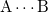dimer is written as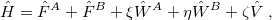(12.9)

where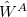and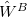are Møller-Plesset fluctuation operators for fragments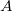and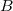, whereas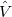consists of the intermolecular Coulomb operators. This part of the perturbation is conveniently expressed as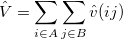(12.10)

with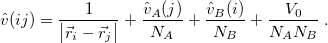(12.11)

The quantity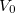is the nuclear interaction energy between the two fragments and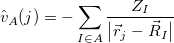(12.12)

describes the interaction of electron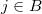with nucleus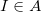.

Starting from a zeroth-order Hamiltonian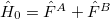and zeroth-order wavefunctions that are direct products of monomer wavefunctions,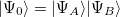, the SAPT approach is based on a symmetrized Rayleigh-Schrödinger perturbation expansion [708, 690] with respect to the perturbation parameters,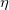, and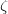in Eq. eq:H_SAPT. The resulting interaction energy can be expressed as[708, 690]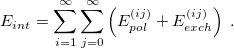(12.13)

Because it makes no sense to treatandat different orders of perturbation theory, there are only two indices in this expansion: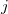for the monomer fluctuations potentials andfor the intermolecular perturbation. The terms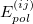are known collectively as the polarization expansion, and these are precisely the same terms that would appear in ordinary Rayleigh-Schrödinger perturbation theory, which is valid when the monomers are well-separated. The polarization expansion contains electrostatic, induction and dispersion interactions, but in the symmetrized  Rayleigh-Schrödinger expansion, each termhas a corresponding exchange term,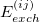, that arises from an antisymmetrizer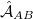that is introduced in order to project away the Pauli-forbidden components of the interaction energy that would otherwise appear .

The version of SAPT that is implemented in Q-Chem assumes that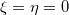, an approach that is usually called SAPT0 . Within the SAPT0 formalism, the interaction energy is formally expressed by the following symmetrized Rayleigh-Schrödinger expansion [708, 690]: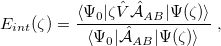(12.14)

The antisymmetrizerin this expression can be written as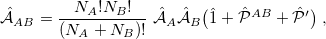(12.15)

where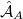and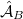are antisymmetrizers for the two monomers and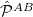is a sum of all one-electron exchange operators between the two monomers. The operator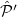in Eq. eq:AB_antisymmetrizer denotes all of the three-electron and higher-order exchanges. This operator is neglected in what is known as the “single-exchange” approximation [708, 690], which is expected to be quite accurate at typical van der Waals and larger intermolecular separations, but sometimes breaks down at smaller intermolecular separations .

Only terms up to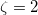in Eq. eq:E(SRS)—that is, second order in the intermolecular interaction—have been implemented in Q-Chem. It is common to relabel these low-order terms in the following way [cf. Eq. eq:triple_pert]: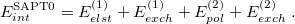(12.16)

The electrostatic part of the first-order energy correction is denoted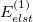and represents the Coulomb interaction between the two monomer electron densities . The quantity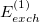is the corresponding first-order (i.e., Hartree-Fock) exchange correction. Explicit formulas for these corrections can be found in Ref. Jeziorski:1993. The second-order term from the polarization expansion, denoted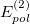in Eq. eq:SAPT_interaction, consists of a dispersion contribution (which arises for the first time at second order) as well as a second-order correction for induction. The latter can be written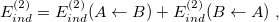(12.17)

where the notation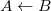, for example, indicates that the frozen charge density ofpolarizes the density of. In detail,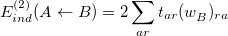(12.18)

where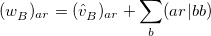(12.19)

and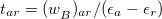. The second term in Eq. eq:E(2)_ind, in whichpolarizes, is obtained by interchanging labels . The second-order dispersion correction has a form reminiscent of the MP2 correlation energy: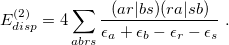(12.20)

The induction and dispersion corrections both have accompanying exchange corrections (exchange-induction and exchange-dispersion) [708, 690].

The similarity between Eq. eq:E(2)_disp and the MP2 correlation energy means that SAPT jobs, like MP2 calculations, can be greatly accelerated using resolution-of-identity (RI) techniques, and an RI version of SAPT is available in Q-Chem. To use it, one must specify an auxiliary basis set. The same ones used for RI-MP2 work equally well for RI-SAPT, but one should always select the auxiliary basis set that is tailored for use with the primary basis of interest, as in the RI-MP2 examples in Section 5.5.1.

It is common to replace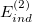and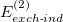in Eq. eq:SAPT_interaction with their “response” (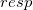) analogues, which are the infinite-order correction for polarization arising from a frozen partner density [708, 690]. Operationally, this substitution involves replacing the second-order induction amplitudes,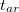in Eq. eq:E(2)_ind_A<-B, with amplitudes obtained from solution of the coupled-perturbed Hartree-Fock equations . (The perturbation is simply the electrostatic potential of the other monomer.) In addition, it is common to correct the SAPT0 binding energy for higher-order polarization effects by adding a correction term of the form [690, 709]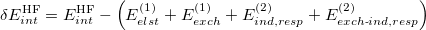(12.21)

to the interaction energy. Here,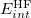is the counterpoise-corrected Hartree-Fock binding energy for. Both the response corrections and the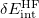correction have been implemented as options in Q-Chem’s implementation of SAPT.

It is tempting to replace Hartree-Fock MOs and eigenvalues in the SAPT0 formulas with their Kohn-Sham counterparts, as a low-cost means of introducing monomer electron correlation. The resulting procedure is known as SAPT(KS) , and does offer an improvement on SAPT0 for some strongly hydrogen-bonded systems . Unfortunately, SAPT(KS) results are generally in poor agreement with benchmark dispersion energies , owing to incorrect asymptotic behavior of approximate exchange-correlation potentials . The dispersion energies can be greatly improved through the use of long-range corrected (LRC) functionals in which the range-separation parameter,, is “tuned” so as to satisfy the condition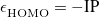, where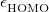is the HOMO energy and “IP" represents the ionization potential . Monomer-specific values of, tuned using the individual monomer IPs, substantially improve SAPT(KS) dispersion energies, though the results are still not of benchmark quality . Other components of the interaction energy, however, can be described quite accurately SAPT(KS) in conjunction with a tuned version of LRC-PBE . Use of monomer-specificvalues is controlled by the variable XPOL_OMEGA in the $rem section, and individual values are entered via an$lrc_omega input section.

Finally, some discussion of basis sets is warranted. Typically, SAPT calculations are performed in the so-called dimer-centered basis set (DCBS) , which means that the combined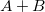basis set is used to calculate the zeroth-order wavefunctions for bothand. This leads to the unusual situation that there are more MOs than basis functions: one set of occupied and virtual MOs for each monomer, both expanded in the same (dimer) AO basis. As an alternative to the DCBS, one might calculate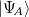using only’s basis functions (similarly for), in which case the SAPT calculation is said to employ the monomer-centered basis set (MCBS) . However, MCBS results are generally of poorer quality. As an efficient alternative to the DCBS, Jacobson and Herbert  introduced a projected (“proj”) basis set, borrowing an idea from dual-basis MP2 calculations . In this approach, the SCF iterations are performed in the MCBS but then Fock matrices for fragmentsandare constructed in the dimer () basis set and “pseudo-canonicalized", meaning that the occupied-occupied and virtual-virtual blocks of these matrices are diagonalized. This procedure does not mix occupied and virtual orbitals, and thus leaves the fragment densities and and zeroth-order fragment energies unchanged. However, it does provide a larger set of virtual orbitals that extend over the partner fragment. This larger virtual space is then used to evaluate the perturbative corrections. All three of these basis options (MCBS, DCBS, and projected basis) are available in Q-Chem.

## 12.8.2 Job Control for SAPT Calculations

Q-Chem’s implementation of SAPT0 was designed from the start as a correction for XPol calculations, a functionality that is described in Section 12.9. As such, a SAPT calculation is requested by setting both of the $rem variable SAPT and XPOL to TRUE. (Alternatively, one may set RISAPT=TRUE to use the RI version of SAPT.) If one wishes to perform a traditional SAPT calculation based on gas-phase SCF monomer wavefunctions rather than XPol monomer wavefunctions, then the$rem variable XPOL_MPOL_ORDER should be set to GAS.

SAPT energy components are printed separately at the end of a SAPT job. If EXCHANGE = HF, then the calculation corresponds to SAPT0, whereas a SAPT(KS) calculation is requested by specifying the desired density functional. [Note that meta-GGAs are not yet available for SAPT(KS) calculations in Q-Chem.] At present, only single-point energies for closed-shell (restricted) calculations are possible. Frozen orbitals are also unavailable.

Researchers who use Q-Chem’s SAPT code are asked to cite Refs. Jacobson:2011 and Herbert:2012.

SAPT
 Requests a SAPT calculation.

TYPE:
 BOOLEAN

DEFAULT:
 FALSE

OPTIONS:
 TRUE Run a SAPT calculation. FALSE Do not run SAPT.

RECOMMENDATION:
 If SAPT is set to TRUE, one should also specify XPOL=TRUE and XPOL_MPOL_ORDER=GAS.

RISAPT
 Requests an RI-SAPT calculation

TYPE:
 BOOLEAN

DEFAULT:
 FALSE

OPTIONS:
 TRUE Compute four-index integrals using the RI approximation. FALSE Do not use RI.

RECOMMENDATION:
 Set to TRUE if an appropriate auxiliary basis set is available, as RI-SAPT is much faster and affords negligible errors (as compared to ordinary SAPT) if the auxiliary basis set is matched to the primary basis set. (The former must be specified using AUX_BASIS.)

SAPT_ORDER
 Selects the order in perturbation theory for a SAPT calculation.

TYPE:
 STRING

DEFAULT:
 SAPT2

OPTIONS:
 SAPT1 First order SAPT. SAPT2 Second order SAPT. ELST First-order Rayleigh-Schrödinger perturbation theory. RSPT Second-order Rayleigh-Schrödinger perturbation theory.

RECOMMENDATION:
 SAPT2 is the most meaningful.

SAPT_EXCHANGE
 Selects the type of first-order exchange that is used in a SAPT calculation.

TYPE:
 STRING

DEFAULT:
 S_SQUARED

OPTIONS:
 S_SQUARED Compute first order exchange in the single-exchange (“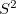") approximation. S_INVERSE Compute the exact first order exchange.

RECOMMENDATION:
 The single-exchange approximation is expected to be adequate except possibly at very short intermolecular distances, and is somewhat faster to compute.

SAPT_BASIS
 Controls the MO basis used for SAPT corrections.

TYPE:
 STRING

DEFAULT:
 MONOMER

OPTIONS:
 MONOMER Monomer-centered basis set (MCBS). DIMER Dimer-centered basis set (DCBS). PROJECTED Projected basis set.

RECOMMENDATION:
 The DCBS is more costly than the MCBS and can only be used with XPOL_MPOL_ORDER=GAS (i.e., it is not available for use with XPol). The PROJECTED choice is an efficient compromise that is available for use with XPol.

SAPT_CPHF
 Requests that the second-order correctionsandbe replaced by their infinite-order “response” analogues,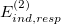and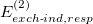.

TYPE:
 BOOLEAN

DEFAULT:
 FALSE

OPTIONS:
 TRUE Evaluate the response corrections and useandFALSE Omit these corrections and useand.

RECOMMENDATION:
 Computing the response corrections requires solving CPHF equations for pair of monomers, which is somewhat expensive but may improve the accuracy when the monomers are polar.

SAPT_DSCF
 Request the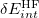correction

TYPE:
 BOOLEAN

DEFAULT:
 FALSE

OPTIONS:
 TRUE Evaluate this correction. FALSE Omit this correction.

RECOMMENDATION:
 Evaluating thecorrection requires an SCF calculation on the entire (super)system. This corrections effectively yields a “Hartree-Fock plus dispersion” estimate of the interaction energy.

SAPT_PRINT
 Controls level of printing in SAPT.

TYPE:
 INTEGER

DEFAULT:
 1

OPTIONS: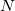Integer print level

RECOMMENDATION:

SAPT_DISP_CORR
 Request an empirical dispersion potential instead of calculating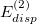and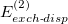directly

TYPE:
 BOOLEAN

DEFAULT:
 FALSE

OPTIONS:
 TRUE Use a dispersion force field. FALSE calculateand.

RECOMMENDATION:
 Using dispersion potentials reduces the scaling from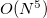to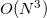with respect to monomer size.

SAPT_DISP_VERSION
 Controls which dispersion potential is used for SAPT

TYPE:
 INTEGER

DEFAULT:
 3

OPTIONS:
 1 Use the “first generation” (+D1) dispersion potentials from Hesselmann [715, 693]. 2 Use the “second generation” (+D2) dispersion potentials from Podeszwa. [716, 694]. 3 Use the “third generation” (+D3) dispersion potentials from Lao .

RECOMMENDATION:
 Use +D3. Whereas +D1 was fit to reproduce binding energies, the +D2 and +D3 potentials were fit directly to dispersion energies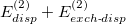computed at the SAPT(DFT) and SAPT2+(3) levels, and performs well for both total binding energies as well as individual energy components [694, 695]. In developing +D3, the training set was expanded to eliminate outliers involvingstacking .

Example 12.275  Example showing a SAPT0 calculation using the RI approximation in a DCBS.

$rem BASIS AUG-CC-PVDZ AUX_BASIS RIMP2-AUG-CC-PVDZ METHOD HF RISAPT TRUE XPOL TRUE XPOL_MPOL_ORDER GAS ! gas-phase monomer wave functions SAPT_BASIS DIMER SYM_IGNORE TRUE$end

$molecule 0 1 -- formamide 0 1 C -2.018649 0.052883 0.000000 O -1.452200 1.143634 0.000000 N -1.407770 -1.142484 0.000000 H -1.964596 -1.977036 0.000000 H -0.387244 -1.207782 0.000000 H -3.117061 -0.013701 0.000000 -- formamide 0 1 C 2.018649 -0.052883 0.000000 O 1.452200 -1.143634 0.000000 N 1.407770 1.142484 0.000000 H 1.964596 1.977036 0.000000 H 0.387244 1.207782 0.000000 H 3.117061 0.013701 0.000000$end